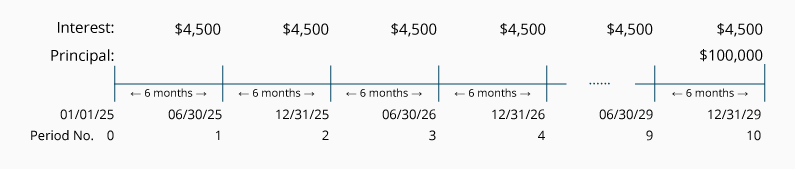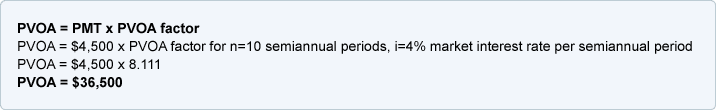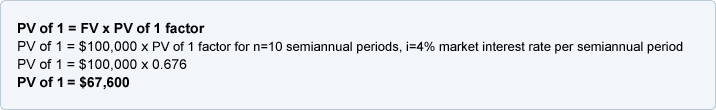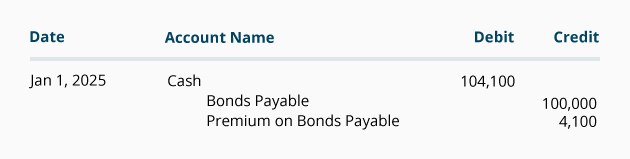# Calculating the Present Value of a 9% Bond in an 8% Market

The present value of a bond is calculated by discounting the bond's future cash payments by the current market interest rate.

In other words, the present value of a bond is the total of:

1. The present value of the semiannual interest payments, PLUS
2. The present value of the principal payment on the date the bond matures.

A 9% \$100,000 bond dated January 1, 2022 and having interest payment dates of June 30 and December 31 of each year for five years will have the following semiannual interest payments and the one-time principal payment:As the timeline indicates, the issuing corporation will pay its bondholders 10 identical interest payments of \$4,500 (\$100,000 x 9% x 6/12 of a year) at the end of each of the 10 semiannual periods, plus a single principal payment of \$100,000 at the end of the 10th six-month period.

The present value (and the market value) of this bond depends on the market interest rate at the time of the calculation. The market interest rate is used to discount both the bond's future interest payments and the principal payment occurring on the maturity date.

#### Here's a Tip

The present value of a bond =

1. The present value of a bond's interest payments, PLUS
2. The present value of a bond's maturity amount.

#### Here's a Tip

Always use the market interest rate to discount the bond's interest payments and maturity amount to their present value.

### 1. Present Value of a Bond's Interest Payments

In our example, there will be interest payments of \$4,500 occurring at the end of every six-month period for a total of 10 six-month or semiannual periods. This series of identical interest payments occurring at the end of equal time periods forms an ordinary annuity.

To calculate the present value of the semiannual interest payments of \$4,500 each, you need to discount the interest payments by the market interest rate for a six-month period. This can be done with computer software, a financial calculator, or a present value of an ordinary annuity (PVOA) table.

We will use present value tables with factors rounded to three decimal places and will round some dollar amounts to the nearest dollar. After you understand the present value concepts and calculations, use computer software or a financial calculator to compute more precise present value amounts.

We will use the Present Value of an Ordinary Annuity (PVOA) Table for our calculations: Click here to open our PVOA Table

Notice that the first column of the PVOA Table has the heading of "n". This column represents the number of identical payments and periods in the ordinary annuity. In computing the present value of a bond's interest payments, "n" will be the number of semiannual interest periods or payments.

The remaining columns are headed by interest rates. These interest rates represent the market interest rate for the period of time represented by "n". In the case of a bond, since "n" refers to the number of semiannual interest periods, you select the column with the market interest rate per semiannual period.

For example, a 5-year bond paying interest semiannually will require you to go down the first column until you reach the row where n = 10. Since n = 10 semiannual periods, you need to go to the column which is headed with the market interest rate per semiannual period. If the market interest rate is 8% per year, you would go to the column with the heading of 4% (8% annual rate divided by 2 six-month periods). Go down the 4% column until you reach the row where n = 10. At the intersection of n = 10, and the interest rate of 4% you will find the appropriate PVOA factor of 8.111.

The factors contained in the PVOA Table represent the present value of a series or stream of \$1 amounts occurring at the end of every period for "n" periods discounted by the market interest rate per period. We will refer to the market interest rates at the top of each column as "i".

#### Here's a Tip

To obtain the proper factor for discounting a bond's interest payments, use the column that has the market's semiannual interest rate "i" in its heading.

Let's use the following formula to compute the present value of the interest payments only as of January 1, 2022 for the bond described above. The amount of the interest payment occurring at the end of each six-month period is represented by "PMT", the number of semiannual periods is represented by "n" and the market interest rate per semiannual period is represented by "i".The present value of \$36,500 tells us that an investor requiring an 8% per year return compounded semiannually would be willing to invest \$36,500 on January 1, 2022 in return for 10 semiannual payments of \$4,500 each—with the first payment occurring on June 30, 2022. The difference between the 10 future payments of \$4,500 each and the present value of \$36,500 equals \$8,500 (\$45,000 minus \$36,500). This \$8,500 return on an investment of \$36,500 gives the investor an 8% annual return compounded semiannually.

Recap

• Use the market interest rate when discounting a bond's semiannual interest payments.
• Convert the market interest rate per year to a semiannual market interest rate, i.
• Convert the number of years to be the number of semiannual periods, n.
• When using the present value tables, use the semiannual market interest rate (i) and the number of semiannual periods (n).

Recall that this calculation determined the present value of the stream of interest payments. The present value of the maturity amount will be calculated next.

### 2. Present Value of a Bond's Maturity Amount

The second component of a bond's present value is the present value of the principal payment occurring on the bond's maturity date. The principal payment is also referred to as the bond's maturity value or face value.

In our example, there will be a \$100,000 principal payment on the bond's maturity date at the end of the 10th semiannual period. The single amount of \$100,000 will need to be discounted to its present value as of January 1, 2022.

To calculate the present value of the single maturity amount, you discount the \$100,000 by the semiannual market interest rate. We will use the Present Value of 1 Table (PV of 1 Table) for our calculations.

Notice that the first column of the PV of 1 Table has the heading of "n". This column represents the number of identical periods that interest will be compounded. In the case of a bond, "n" is the number of semiannual interest periods or payments. In other words, the number of periods for discounting the maturity amount is the same number of periods used for discounting the interest payments.

The remaining columns of the PV of 1 Table are headed by interest rates. The interest rate represents the market interest rate for the period of time represented by "n". In the case of a bond, since "n" refers to the number of semiannual interest periods, you select the column with the market interest rate per semiannual period.

For example, a 5-year bond paying interest semiannually will require you to go down the first column until you reach the row where n = 10. Since n = 10 semiannual periods, you need to go to the column which is headed with the market interest rate per semiannual period. If the market interest rate is 8% per year, you would go to the column with the heading of 4% (8% annual rate divided by 2 six-month periods). Go down the 4% column until you reach the row where n = 10. At the intersection of n = 10, and the interest rate of 4%, you will find the PV of 1 factor of 0.676.

The factors contained in the PV of 1 Table represent the present value of a single payment of \$1 occurring at the end of the period "n" discounted by the market interest rate per period, which will be noted as "i".

#### Here's a Tip

To obtain the proper factor for discounting a bond's maturity value, use the PV of 1 Table and use the same "n" and "i" that you used for discounting the semiannual interest payments.

Let's use the following formula to compute the present value of the maturity amount only of the bond described above. The maturity amount, which occurs at the end of the 10th six-month period, is represented by "FV" .The present value of \$67,600 tells us that an investor requiring an 8% per year return compounded semiannually would be willing to invest \$67,600 in return for a single receipt of \$100,000 at the end of 10 semiannual periods of time. The difference between the present value of \$67,600 and the single future principal payment of \$100,000 is \$32,400. This \$32,400 return on an investment of \$67,600 gives the investor an 8% annual return compounded semiannually.

Recap

When calculating the present value of the maturity amount...

Use the semiannual market interest rate (i) and the number of semiannual periods (n) that were used to calculate the present value of the interest payments.

### Combining the Present Value of a Bond's Interest and Maturity Amounts

Recall that the present value of a bond consisted of:

1. The present value of a bond's interest payments, PLUS
2. The present value of a bond's maturity amount.

The present value of the bond in our example is \$36,500 + \$67,600 = \$104,100.

The bond's total present value of \$104,100 should approximate the bond's market value.

It is reasonable that a bond promising to pay 9% interest will sell for more than its face value when the market is expecting to earn only 8% interest. In other words, the 9% bond will be paying \$500 more semiannually than the bond market is expecting (\$4,500 vs. \$4,000). If investors will be receiving an additional \$500 semiannually for 10 semiannual periods, they are willing to pay \$4,100 more than the bond's face amount of \$100,000. The \$4,100 more than the bond's face amount is referred to as Premium on Bonds Payable, Bond Premium, Unamortized Bond Premium, or Premium.

The journal entry to record a \$100,000 bond that was issued for \$104,100 on January 1, 2022 is: